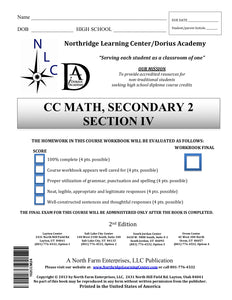# CC Math, Secondary 2, Section IV

Regular price
\$56.50
Regular price
Sale price
\$56.50
Unit price
per
Availability
Sold out

Common Core Mathematics, Secondary II 07-08-0000-100

In the second year of the Common Core Math series, students will build upon knowledge of foundational math skills to learn to solve increasingly complex problems. This course begins with the introduction of exponents and covers key concepts such as polygon perimeter and area calculations, geometric theorems and postulates, techniques for solving quadratic equations and an introduction to basic trigonometry.

d apply similarity in right triangles to understand right triangle trigonometry, with particular attention to special right triangles and the Pythagorean theorem. Students will develop facility with geometric proof. They use what they know about congruence and similarity to prove theorems involving lines, angles, triangles, and other polygons. They explore a variety of formats for writing proofs. Students will prove basic theorems about circles, such as a tangent line is perpendicular to a radius, inscribed angle theorem, and theorems about chords, secants, and tangents dealing with segment lengths and angle measures. They will study relationships among segments on chords, secants, and tangents as an application of similarity. In the Cartesian coordinate system, students will use the distance formula to write the equation of a circle when given the radius and the coordinates of its center, and the equation of a parabola with vertical axis when given an equation of its directrix and the coordinates of its focus. Given an equation of a circle, they will draw the graph in the coordinate plane, and apply techniques for solving quadratic equations to determine intersections between lines and circles or a parabola and between two circles. Students will develop informal arguments justifying common formulas for circumference, area, and volume of geometric objects, especially those related to circles.

30-Day Extension - Click Here